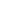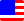Documents  >  Tutorial  >  Priority 6
Priority 6 operators

It describes the following operators with priority 6.

 < x < y Less than operator <= x <= y Less than or equal operator > x > y Greater than operator >= x >= y Greater than equal operator

They are binary comparison operators. The magnitude relation of the left and right operands is compared and the result is returned by the bool class.

The operand must be one of the int, long, or real classes. These classes can be mixed in the left and right operands.

Less than operator

Less than or equal operator

Greater than operator

Greater than equal operator

Less than operator

Less than operator returns true if the left operand is less than the right operand. Otherwise, it returns false.

bool result0 = 10 < 11; //The result is true;

bool result1 = 10 < 10; //The result is false;

bool result2 = 11 < 10; //The result is false;

If operands return the proxy class, the entity of the proxy is automatically used. The result is the same as calling the Entity getter of the proxy class.

int i = 10;

real r = 10.5;

proxy pro = new proxy(i);

proxy pro2 = new proxy(r);

bool b = pro2 < pro; // b is false.

Less than or equal operator

Less than or equal operator returns true if the left operand is less than or equal to the right operand. Otherwise, it returns false.

bool result0 = 10 <= 11; //The result is true;

bool result1 = 10 <= 10; //The result is true;

bool result2 = 11 <= 10; //The result is false;

If operands return the proxy class, the entity of the proxy is automatically used. The result is the same as calling the Entity getter of the proxy class.

int i = 10;

real r = 10.5;

proxy pro = new proxy(i);

proxy pro2 = new proxy(r);

bool b = pro2 < pro; // b is false.

Greater than operator

Greater than operator returns true if the left operand is greater than the right operand. Otherwise, it returns false.

bool result0 = 10 > 11; //The result is false;

bool result1 = 10 > 10; //The result is false;

bool result2 = 11 > 10; //The result is true;

If operands return the proxy class, the entity of the proxy is automatically used. The result is the same as calling the Entity getter of the proxy class.

int i = 10;

real r = 10.5;

proxy pro = new proxy(i);

proxy pro2 = new proxy(r);

bool b = pro2 > pro; // b is true.

Greater than equal operator

Greater than or equal operator returns true if the left operand is greater than or equal to the right operand. Otherwise, it returns false.

bool result0 = 10 >= 11; //The result is false;

bool result1 = 10 >= 10; //The result is true;

bool result2 = 11 >= 10; //The result is true;

If operands return the proxy class, the entity of the proxy is automatically used. The result is the same as calling the Entity getter of the proxy class.

int i = 10;

real r = 10.5;

proxy pro = new proxy(i);

proxy pro2 = new proxy(r);

bool b = pro2 > pro; // b is true.